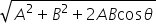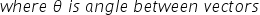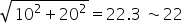science
Easy

Question

# Find the resultant of the vector if two orthogonal vectors are of magnitude 10 m and 20 m.

## 22563541Hint:

## The correct answer is: 22

### Let R be Resultant and R=here they are orthogonal so angle = 90 degreeand cos90 =0 so R=#### With Turito Foundation.#### Get an Expert Advice From Turito.